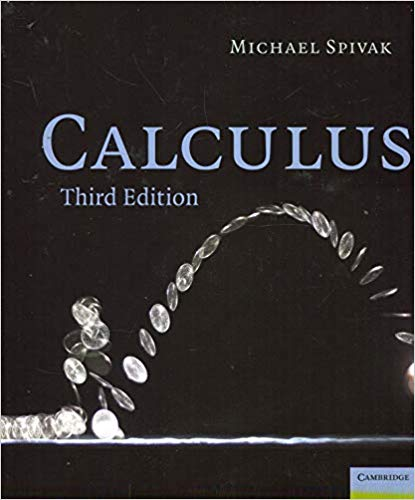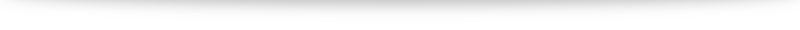# My introduction to Spivak: Calculus

“Analysis” is one of the major fields of mathematics and the path a typical student of mathematics goes through in this field goes something like this:

• “Calculus”: This is where we are more concerned about the techniques of differentiation and integration. In the American context this is typically done in AP classes + at lower level undergraduate courses. In Singapore we start this journey in Additional Mathematics at the “O” levels where differentiation (techniques and applications) are covered extensively with a brief introduction into integration techniques. At the “A” levels we delve deeper into further integration techniques and start touching on some interesting applications of calculus through areas and volumes, the Maclaurin series and differential equations. At university this is usually taken further through an introduction to limits and further courses on differential equations (both ordinary and partial). This is also where many math-adjacent courses (engineering and sciences) end in this endeavor.
• “Analysis”: The concept of proofs is where the (pure) mathematics syllabus start to deviate from their applied mathematics, science and engineering contemporaries. And this is where I feel we start moving from “Calculus” into “Analysis”, where the proof of equations and theorems start to take more importance compared to the use and application of them. In a sense, we re-learn what we have started taken for granted in our earlier study and place them on solid foundation. Analysis I typically takes a student through limits and the epsilon-delta formulation, sequences and differentiation, Analysis II goes into the (Riemann) integral while Analysis III goes into the link between the two: The Fundamental Theorem of Calculus. With interesting detours along the way (like the Taylor series) in between.
• “Measure Theory and the Lebesgue integral”: At the upper undergraduate and beginning graduate level we move on from the Riemann integral to the Lebesgue integral, with a whole big idea of measure theory supporting the approach. This leads to all sorts of related topics such as topology, functional analysis, probability theory and more!

As I went through my own path (and coming from a engineering background to graduate work in math) I am often in awe of this progression of ideas. Unfortunately, the pace of school work, having to complete each course within 10-12 weeks with a final examination at the end means I sometimes did not have the time to fully appreciate some of the ideas and hardly took any interesting looking detours. Having needed a few courses around measure theory before I truly understood and appreciated it, I feel I could benefit from a slower but deeper delve into the more “basic” analysis portion of my study.

I’ve read many good reviews of Spivak’s Calculus (the book title being a bit of a misnomer: it is definitely a book aimed at the “analysis” part rather than the “calculus” part as I’ve described above) and am eager to give it a whirl. Chapters I’m especially looking forward to include the proof that $\pi$ is irrational, $e$ is transcendental, how he defines the logarithm and exponential function and the construction of the real numbers (plus a proof in its uniqueness). As I work through the book I’m looking to use this as a blog to aid my understanding of the material.

I’m using the third edition, though I understand the 4th edition is out (amazon link to the third edition: cover photo credits). Let’s enjoy this journey together then!